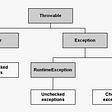# Dual Pivot Quick Sort : Java’s default sorting algorithm for primitive types.

What happens when we run Arrays.sort() in Java? which sorting algorithm Java uses in background?

Since Java 7 release back in 2011, default sorting algorithm used is DualPivotQuickSort which is an enhancement over classic quick sort algorithm.

Dual pivot quick sort is a combination of insertion sort and quick sort. Insertion sort has faster runtime when the number of elements to be sorted is small, Double pivot quick sort uses this fact thus when the number of elements are <= 47 Java performs insertion sort under the hood.

When input size array is larger than 47 Java uses Double pivot quick sort. As the name suggests DualPivotQuickSort algorithm picks 2 pivot instead of 1. Unlike quick sort in which we partition the array around 1 pivot, we partition the array in three parts around 2 pivots.

1st sub array : items < LP 2nd sub array : LP <= items <= RP

3rd sub array =: items >= RP

Algorithm

LP: Left Pivot
RP: Right Pivot

1.) Left most item in an array is taken as the left pivot and Right most item as the right pivot. 2.) Swap Left pivot with Right Pivot if LP > RP

3.) Partition array in three sub-arrays around left and right pivot.

Once partition is done we perform above 3 steps recursively on three sub-array.

Let’s walk through an example-:

No need to swap pivots in above image since LP < RP.
Now we partition the array following below schemes.

1st sub array : items < LP 2nd sub array : LP <= items <= RP

3rd sub array =: items >= RP

Now we have 3 sub-array over which we’ll perform same steps as above. Since first two arrays have only 2 items, one will become left pivot and other will become right pivot. We’ll swap left pivot with right pivot if left pivot is greater than right pivot which is not the case for first two sub- arrays.

For the third sub-array as we can see in below image left pivot is greater than right pivot thus we’ll swap them.

Swapping pivots.

We don’t have any more elements in the array that we can partition further.

References

Conclusion
Similarly Python uses Timsort which is a combination of insertion sort and merge sort. I’d like to read and write about different other variation of quick sort in next few days.

Stay tuned:)

Originally published at dev.to.

--

--

--

## More from AWDESH

Learner, Philomath. Engineer by choice, humorous by nature. Big fan of technology.

Love podcasts or audiobooks? Learn on the go with our new app.## AWDESH

Learner, Philomath. Engineer by choice, humorous by nature. Big fan of technology.

## Difference between throw & throws in Java?